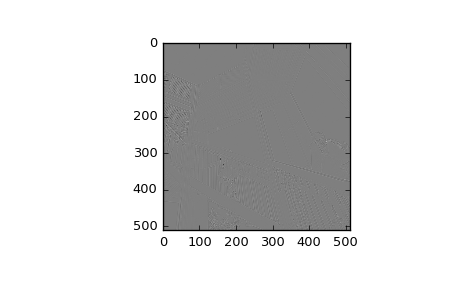# scipy.ndimage.laplace¶

scipy.ndimage.laplace(input, output=None, mode='reflect', cval=0.0)[source]

N-dimensional Laplace filter based on approximate second derivatives.

Parameters: input : array_like Input array to filter. output : array, optional The output parameter passes an array in which to store the filter output. mode : {‘reflect’, ‘constant’, ‘nearest’, ‘mirror’, ‘wrap’}, optional The mode parameter determines how the array borders are handled, where cval is the value when mode is equal to ‘constant’. Default is ‘reflect’ cval : scalar, optional Value to fill past edges of input if mode is ‘constant’. Default is 0.0

Examples

>>> from scipy import ndimage, misc
>>> import matplotlib.pyplot as plt
>>> ascent = misc.ascent()
>>> result = ndimage.laplace(ascent)
>>> plt.gray()  # show the filtered result in grayscale
>>> plt.imshow(result)#### Previous topic

scipy.ndimage.generic_laplace

#### Next topic

scipy.ndimage.maximum_filter